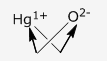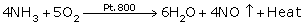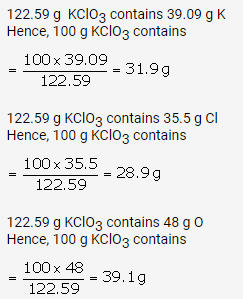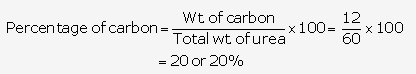## Selina Concise Chemistry Class 9 ICSE Solutions The Language of Chemistry

APlusTopper.com provides step by step solutions for Selina Concise ICSE Solutions for Class 9 Chemistry Chapter 1 Language of Chemistry. You can download the Selina Concise Chemistry ICSE Solutions for Class 9 with Free PDF download option. Selina Publishers Concise Chemistry for Class 9 ICSE Solutions all questions are solved and explained by expert teachers as per ICSE board guidelines.

Selina ICSE Solutions for Class 9 Chemistry Chapter 4 The Language of Chemistry

Page No: 8

Question 1.
What is a symbol? What information does it convey?
Solution:
A symbol is the short form which stands for the atom of a specific element or the abbreviations used for the names of elements.

1. It represents a specific element.
2. It represents one atom of an element.
3. A symbol represents how many atoms are present in its one gram (gm) atom.
4. It represents the number of times an atom is heavier than one atomic mass unit (amu) taken as a standard.
Question 2
Why is the symbol S for sulphur, but Na for sodium and Si for silicon?
Solution:
In most cases, the first letter of the name of the element is taken as the symbol for that element and written in capitals (e.g. for sulphur, we use the symbol S). In cases where the first letter has already been adopted, we use a symbol derived from the Latin name (e.g. for sodium/Natrium, we use the symbol Na). In some cases, we use the initial letter in capital together with a small letter from its name (e.g. for silicon, we use the symbol Si).
Question 3.
Write the full form of IUPAC. Name the elements represented by the following symbols:
Au, Pb, Sn, Hg
Solution:
The full form of IUPAC is International Union of Pure and Applied Chemistry.
Names of the elements:
Au – Gold
Sn – Tin
Hg – Mercury
Question 4.
If the symbol for Cobalt, Co, were written as CO, what would be wrong with it?
Solution:
Co stands for Cobalt. If we write CO, then it would mean that it is a compound containing two non-metal ions, i.e. carbon and oxygen, which forms carbon monoxide gas.

Question 5(d).
2H2
Solution:
(a) H stands for one atom of hydrogen.
(b) H2 stands for one molecule of hydrogen.
(c) 2H stands for two atoms of hydrogen.

Question 6.
What is meant by atomicity? Name the diatomic element.
Solution:
The number of atoms of an element that join together to form a molecule of that element is known as its atomicity. Diatomic molecules: H2, O2, N2, Cl2
Question 7(a).
Explain the terms ‘valency‘ and ‘variable valency‘.
Solution:
1. Valency of Na is +1 because it can lose one electron.
2. Valency of O is -2 because it can accept two electrons.

Variable valency: It is the combining capacity of an element in which the metal loses more electrons from a shell next to a valence shell in addition to electrons of the valence shell.

Question 7(b).
How are the elements with variable valency named? Explain with an example.
Solution:
If an element exhibits two different positive valencies, then
1. for the lower valency, use the suffix -OUS at the end of the name of the metal
2. for the higher valency, use the suffix -IC at the end of the name of the metal.

Example:

 Element Lower valency Higher valency Ferrum (Iron) Ferrous (Fe2+) Ferric (Fe3+)

Question 8.
Give the formula and valency of:

1. aluminate ………………… .
2. chromate ………….…….. .
3. aluminium ………………. .
4. cupric  ………………… .

Solution:

 Name Formula Valency a. Aluminate AlO2 -2 b. Chromate CrO4 -2 c. Aluminium Al +3 d. Cupric Cu +2

Question 9.b
What is the significance of formula?
Solution:
Chemical formula: The chemical formula of a substance (element or compound) is a symbolic representation of the actual number of atoms present in one molecule of that substance.
It also indicates the fixed proportion by weight in which atoms combine.
Rules:
(i) The positive and negative radicals are written side by side (+ve first) with their charge as a superscript on the right side.
(ii) Charges are then interchanged and written as a subscript.
(iii) The final formula is written without the sign of charge, e.g. Hg2O

1. Hg1+O2-
2.3. Hg2O

Question 10(a).
What do you understand by the following terms?
Solution:
Examples: Cl, O2-

Question 10(b).
What do you understand by the following terms? Basic radical
Solution:
Examples: K+, Na+

Question 11.

Match the following:

 Compound Formula (a) Boric acid i. NaOH (b) Phosphoric acid ii. SiO2 (c) Nitrous acid iii. Na2CO3 (d) Nitric acid iv. KOH (e) Sulphurous acid v. CaCO3 (f) Sulphuric acid vi. NaHCO3 (g) Hydrochloric acid vii. H2S (h) Silica (sand) viii. H2O (i) Caustic soda (sodium hydroxide) ix. PH3 (j) Caustic potash (potassium hydroxide) x. CH4 (k) Washing soda(sodium carbonate) xi. NH3 (l) Baking soda(sodium bicarbonate) xii. HCl (m) Lime stone.(calcium carbonate) xiii. H2SO3 (n) Water xiv. HNO3 (o) Hydrogen sulphide xv. HNO2 (p) Ammonia xvi. H3BO3 (q) Phosphine xvii. H3PO4 (r) Methane xviii. H2SO4

Solution:

 Compound Formula (Ans) (a) Boric acid xvi. H3BO3 (b) Phosphoric acid xvii. H3PO4 (c) Nitrous acid xv. HNO2 (d) Nitric acid xiv. HNO3 (e) Sulphurous acid xiii. H2SO3 (f) Sulphuric acid xviii. H2SO4 (g) (a) Hydrochloric acid xii. HCl (h) Silica (sand) ii. SiO2 (i) Caustic soda (sodium hydroxide) i. NaOH (j) Caustic potash (potassium hydroxide) iv. KOH (k) Washing soda (sodium carbonate) iii. Na2CO3 (l) Baking soda (sodium bicarbonate) vi. NaHCO3 (m) Lime stone (calcium carbonate) v. CaCO3 (n) Water viii. H2O (o) Hydrogen sulphide vii. H2S (p) Ammonia xi. NH3 (q) Phosphine ix. PH3 (r) Methane x. CH4

Question 12.
Select the basic and acidic radicals in the following compounds.

1. MgSO4
2. (NH4)2SO4
3. Al2(SO4)3
4. ZnCO3
5. Mg(OH)2

Solution:

 Acidic radical Basic radical MgSO4 SO4– Mg+ (NH4)2SO4 SO4– NH4+ Al2(SO4)3 SO4– Al3+ ZnCO3 CO3– Zn2+ Mg(OH)2 OH– Mg2+
Question 13.
Write chemical formula of the sulphate of Aluminium, Ammonium and Zinc.
Solution:
Valencies of aluminium, ammonium and zinc are 3, 1 and 2, respectively.
The valency of sulphate is 2.
Hence, chemical formulae of the sulphates of aluminium, ammonium and zinc are Al2(SO4)3, (NH4)2SOand ZnSO4.
Question 14.
The valency of an element A is 3 and that of element B is 2. Write the formula of the compound formed by the combination of A and B
Solution:
Formula of the compound = A2B3

Question 15.
Write the chemical names of the following compounds:

1. Ca3(PO4)2
2. K2CO3
3. K2MnO4
4. Mn3(BO3)2
5. Mg(HCO3)2
6. Na4Fe(CN)6
7. Ba(ClO3)2
8. Ag2SO3
9. (CH3COO)2Pb
10. Na2SiO3

Solution:
Chemical names of compounds:

1. Ca3(PO4)2 – Calcium phosphate
2. K2CO3 – Potassium carbonate
3. K2MnO– Potassium manganate
4. Mn3(BO3)2 – Manganese (II) borate
5. Mg(HCO3)2 – Magnesium hydrogen carbonate
6. Na4Fe(CN)6 – Sodium ferrocyanide
7. Ba(ClO3)2 – Barium chlorate
8. Ag2SO3 – Silver sulphite
10. Na2SiO3 – Sodium silicate

Question 16
Write the basic radicals and acidic radicals of the following and then write the chemical formulae of these compounds.

1. Barium sulphate
2. Bismuth nitrate
3. Calcium bromide
4. Ferrous sulphide
5. Chromium sulphate
6. Calcium silicate
7. Potassium ferrocyanide
8. Stannic oxide
9. Magnesium phosphate
10. Sodium zincate
11. Stannic phosphate
12. Sodium thiosulphate
13. Potassium manganate
14. Nickel bisulphate

Solution:

 Compounds Acidic radical Basic radical Chemical formulae Barium sulphate SO42- Ba2+ BaSO4 Bismuth nitrate NO3– Bi3+ Bi(NO3)3 Calcium bromide Br– Ca2+ CaBr2 Ferrous sulphide S2- Fe2+ FeS Chromium sulphate SO42- Cr3+ Cr2(SO4)3 Calcium silicate SiO42- Ca2+ Ca2SiO4 Potassium ferrocyanide [Fe(CN)6]4- K1+ K4[Fe(CN)6] Stannic oxide O2- Sn2+ SnO2 Magnesium phosphate (PO4)3- Mg2+ Mg3(PO4)2 Sodium zincate ZnO2- Na1+ Na2ZnO2 Stannic phosphate (PO4)3- Sn4+ Sn3(PO4)4 Sodium thiosulphate (S2O3)2- Na1+ Na2S2O3 Potassium manganate MnO42- K1+ K2MnO4 Nickel bisulphate HSO41- Ni3+ Ni(HSO4)3

Question 16.
Give the names of the following compounds.

1. NaClO
2. NaClO2
3. NaClO3
4. NaClO4

Solution:

1. NaClO – Sodium hypochlorite
2. NaClO2 – Sodium chlorite
3. NaClO3 – Sodium chlorate
4. NaClO4 – Sodium perchlorate

Question 18(a).
Complete the following statements by selecting the correct option :
The formula of a compound represents
i. an atom
ii. a particle
iii. a molecule
iv. a combination
Solution:
iii. The formula of a compound represents a molecule.

Question 18(b).
Complete the following statements by selecting the correct option :
The correct formula of aluminium oxide is
i. AlO3
ii. AlO2
iii. Al2O3
Solution:
iii. The correct formula of aluminium oxide is Al2O3.

Question 18(c).
Complete the following statements by selecting the correct option :
The valency of nitrogen in nitrogen dioxide (NO2) is
i. one
ii. two
iii. three
iv. four
Solution:
iv. The valency of nitrogen in nitrogen dioxide (NO2) is four.

Page No: 13

Question 1.
Balance the following equations:
1. Fe + H2O → Fe3O4 + H2
2. Ca + N2 → Ca3N2
3. Zn + KOH → K2ZnO2 + H2
4. Fe2O3 + CO → Fe + CO2
5. PbO + NH3 → Pb + H2O + N2
6. Pb3O4 → PbO + O2
7. PbS + O2 → PbO + SO2
8. S + H2SO→ SO2 + H2O
9. S + HNO3 → H2SO4 + NO2 + H2O
10. MnO2 + HCl → MnCl2 + H2O + Cl2
11. C + H2SO4 → CO2 + H2O + SO2
12. KOH + Cl2 → KCl + KClO + H2O
13. NO2 +H2O → HNO2 + HNO3
14.  Pb3O4 + HCl → PbCl2 + H2O + Cl2
15.  H2O + Cl2 → HCl + O2
16.  NaHCO3 → Na2CO3 + H2O + CO2
17. HNO3 + H2S → NO2 + H2O + S
18. P + HNO3 → NO2 + H2O + H3PO4
19. Zn + HNO3  → Zn(NO3)2 + H2O + NO2

Solution:
Balanced chemical equations:

1. 3Fe + 4H2O → Fe3O4 + 4H2
2. 3Ca + N2 → Ca3N2
3. Zn + 2KOH → K2ZnO2 + H2
4. Fe2O3 + 3CO → 2Fe + 3CO2
5. 3PbO + 2NH3 → 3Pb + 3H2O + N2
6. 2Pb3O4 → 6PbO + O2
7. 2PbS + 3O2 → 2PbO + 2SO2
8. S + 2H2SO→ 3SO2 + 2H2O
9. S + 6HNO3 → H2SO4 + 6NO2 + 2H2O
10. MnO2 + 4HCl → MnCl2 + 2H2O + Cl2
11. C + 2H2SO4 → CO2 + H2O + SO2
12. 2KOH + Cl2 → KCl + KClO + H2O
13. 2NO2 + H2O → HNO2 + HNO3
14. Pb3O4 + 8HCl → 3PbCl2 + 4H2O + Cl2
15. 2H2O + 2Cl2 → 4HCl + O2
16. 2NaHCO3 → Na2CO3 + H2O + CO2
17. 2HNO3 + H2S → 2NO2 + 2H2O + S
18. P + 5HNO3 → 5NO2 + H2O + H3PO4
19. Zn + 4HNO3  → Zn(NO3)2 + 2H2O + 2NO2

Page No: 17

Question 1.
Fill in the blanks:

1. Dalton used symbol _____ for oxygen _____ for hydrogen.
2. Symbol represents _____ atom(s) of an element.
3. Symbolic expression for a molecule is called _____.  .
5. Valency of carbon in CH4 is _____ , in C2H_____, in C2H4 ___ and in C2H2 is ____.
6. Valency of Iron in FeCl2 is _____  and in FeCl3 it is ____ .
7. Formula of iron (ill) carbonate is _____ .

Solution:

1. Dalton used symbol [O] for oxygen,[H] for hydrogen.
2. Symbol represents gram atom(s) of an element.
3. Symbolic expression for a molecule is called molecular formula.
4. Sodium chloride has two radicals. Sodium is a basic radical, while chloride is an acid radical.
5. Valency of carbon in CH4 is 4, in C2H64, in C2H44 and in C2H2 is 4.
6. Valency of iron in FeCl2 is 2 and in FeCl3 it is 3.
7. Formula of iron (III) carbonate is Fe2[CO3]3.

Question 2.
Complete the following table.

 Acid Radicals Basic Radicals Chloride Nitrate Sulphate Carbonate Hydroxide Phosphate Magnesium MgCl2 Mg(NO3)2 MgSO4 MgCO3 Mg(OH)2 Mg3(PO4)2 Sodium Zinc Silver Ammonium Calcium Iron (II) Potassium

Solution:Question 3.
Sodium chloride reacts with silver nitrate to produce silver chloride and sodium nitrate

1. Write the equation.
2. Check whether it is balanced, if not balance it.
3. Find the weights of reactants and products.
4. State the law which this equation satisfies.

Solution:
(a) NaCl+ AgNO3 → NaNO3 + AgCl↓
(b) It is a balanced equation.
(c) Weights of reactants:NaCl – 58.44, AgNO3 – 169.87
Weights of products: NaNO3 – 84.99, AgCl – 143.32
NaCl + AgNO3 → NaNO + AgCl
(23+35.5) + (108+14+48) → (23+14+48) + (108+35.5)
58.5 + 170 → 85 + 143.5
228.5 g → 228.5 g
(d) Law of conservation of mass: Matter is neither created nor destroyed in the course of a chemical reaction.

Question 4(a).
What information does the following chemical equation convey? Zn + H2SO4 → ZnSO4+ H2
Solution:
(a) This equation conveys the following information:
1. The actual result of a chemical change.
2. Substances take part in a reaction, and substances are formed as a result of the reaction.
3. Here, one molecule of zinc and one molecule of sulphuric acid react to give one molecule of zinc sulphate and one molecule of hydrogen.
4. Composition of respective molecules, i.e. one molecule of sulphuric acid contains two atoms of hydrogen, one atom of sulphur and four atoms of oxygen.
5. Relative molecular masses of different substances, i.e. molecular mass of
Zn = 65
H2SO4 = (2+32+64) = 98
ZnSO= (65+32+64) = 161
H2 = 2
6. 22.4 litres of hydrogen are formed at STP.
Question 4(b).
What information do the following chemical equations convey? Mg + 2HCl → MgCl2+ H2
Solution:
(b) This equation conveys the following information:
1. Magnesium reacts with hydrochloric acid to form magnesium chloride and hydrogen gas.
2. 24 g of magnesium reacts with 2(1 + 35.5) = 73 g of hydrochloric acid to produce (24 + 71), i.e. 95 g of magnesium chloride.
3. Hydrogen produced at STP is 22.4 litres.
Question 5(a).
What are polyatomic ions? Give two examples.
Solution:
(a) A poly-atomic ion is a charged ion composed of two or more atoms covalently bounded that can be carbonate (CO32-) and sulphate (SO42-)
Question 5(b).
Name the fundamental law that is involved in every equation.
Solution:
(b) The fundamental laws which are involved in every equation are:
1. A chemical equation consists of formulae of reactants connected by plus sign (+) and arrow (→) followed by the formulae of products connected by plus sign (+).
2. The sign of an arrow (→) is to read ‘to form’. It also shows the direction in which reaction is predominant.
Question 6(a).
What is the valency of : fluorine in CaF2
Solution:
(a) Valency of fluorine in CaFis -1.
Question 6(b).
What is the valency of :
sulphur in SF6
Solution:
(b) Valency of sulphur in SF6 is -6.

Question 6(c).

What is the valency of :

phosphorus in PH3
Solution:
(c) Valency of phosphorus in PH3 is +3.

Question 6(d).
What is the valency of : carbon in CH4
Solution:
(d) Valency of carbon in CH4 is +4.
Question 6(e).
What is the valency of :
nitrogen in the following compounds:
(i) N2O3 (ii) N2O5 (iii) NO2 (iv) NO
Solution:
(e) Valency of nitrogen in the given compounds:
1. N2O = N is +3
2. N2O= N is +5
3. NO2 = N is +4
4. NO = N is +2
Question 7.
Why should an equation be balanced? Explain with the help of a simple equation.
Solution:
According to law of conservation of mass, “matter can neither be created nor be destroyed in a chemical reaction”. This is possible only, if total number of atoms on the reactants side is equals to total number of atoms on products side. Thus, a chemical reaction should be always balanced.
Let us consider an example,
Fe + H2O → Fe3O4 + H2
In this equation number of atoms on both sides is not the same, the equation is not balanced.
The balanced form of this equation is given by,
3Fe + 4H2O  → Fe3O4 + 4H2
Question 8(a).
Write the balanced chemical equations of the following reactions. sodium hydroxide + sulphuric acid → sodium sulphate + water
Solution:
2NaOH + H2SO4 → Na2SO4 + 2H2O

Question 8(b).
Write the balanced chemical equations of the following reactions. potassium bicarbonate + sulphuric acid → potassium sulphate + carbon dioxide + water
Solution:
2KHCO3 + H2SO4 → K2SO4 + 2CO2 + 2H2O

Question 8(c).
Write the balanced chemical equations of the following reactions. iron + sulphuric acid → ferrous sulphate + hydrogen.
Solution:
Fe + H2SO4 → FeSO4 + H2

Question 8(d).
Write the balanced chemical equations of the following reactions. chlorine + sulphur dioxide + water →  sulphuric acid + hydrogen chloride
Solution:
Cl2 + SO2 + 2H2O → H2SO4 + 2HCl

Question 8(e).
Write the balanced chemical equations of the following reactions. silver nitrate → silver + nitrogen dioxide + oxygen”
Solution:
2AgNO3 → 2Ag + 2NO2 + O2

Question 8(f).
Write the balanced chemical equations of the following reactions.
copper + nitric acid → copper nitrate + nitric oxide + water
Solution:
3Cu + 8HNO3 → 3Cu(NO3)2 + 2NO + 4H2O

Question 8(g).
Write the balanced chemical equations of the following reactions.
ammonia + oxygen → nitric oxide + water
Solution:Question 8(h).
Write the balanced chemical equations of the following reactions.
barium chloride + sulphuric acid → barium sulphate + hydrochloric acid
Solution:
BaCl2 + H2SO4 → BaSO4 + 2HCl

Question 8(i).
Write the balanced chemical equations of the following reactions.
zinc sulphide + oxygen → zinc oxide + sulphur dioxide
Solution:
2ZnS + 3O2 → 2ZnO + 2SO2

Question 8(j).
Write the balanced chemical equations of the following reactions.
aluminium carbide + water → aluminium hydroxide + methane
Solution:
Al4C3 + 12H2O → 4Al(OH)3 + 3CH4

Question 8(k).
Write the balanced chemical equations of the following reactions.
iron pyrites(FeS2) + oxygen → ferric oxide + sulphur dioxide
Solution:
4FeS2 + 11O2 → 2Fe2O3 + 8SO2

Question 8(l).
Write the balanced chemical equations of the following reactions.
potassium permanganate + hydrochloric acid → potassium chloride + manganese chloride + chlorine + water
Solution:
2KMnO4 + HCl → 2KCl + 2MnCl2 + 5Cl2 + 8H2O

Question 8(m).
Write the balanced chemical equations of the following reactions.
aluminium sulphate + sodium hydroxide → sodium sulphate + sodium meta aluminate + water.
Solution:
Al2(SO4)+ 8NaOH → 3Na2SO4 + 2NaAlO2 + 4H2O

Question 8(n).
Write the balanced chemical equations of the following reactions.
aluminium + sodium hydroxide + water → sodium meta aluminate + hydrogen
Solution:
2Al + 2NaOH + 2H2O → 2NaAlO2 + 3H2

Question 8(o).
Write the balanced chemical equations of the following reactions.
potassium dichromate + sulphuric acid → potassium sulphate + chromium sulphate + water + oxygen.
Solution:
2K2Cr2O7 + 8H2SO4 → 2K2SO4 + 2Cr2(SO4)3 + 8H2O + 3O2

Question 8(p).
Write the balanced chemical equations of the following reactions.
potassium dichromate + hydrochloric acid → Potassium chloride + chromium chloride + water + chlorine
Solution:
K2Cr2O7 + 14HCl → 2KCl + 2CrCl3 + 7H2O + 3Cl2

Question 8(q).
Write the balanced chemical equations of the following reactions.
sulphur + nitric acid → sulphuric acid + nitrogen dioxide + water.
Solution:
S + HNO3 → H2SO4 + NO2 + H2O

Question 8(r).
Write the balanced chemical equations of the following reactions.
sodium chloride + manganese dioxide + sulphuric acid → sodium hydrogen sulphate  + manganese sulphate + water + chlorine.
Solution:
2NaCl + MnO2 + 3H2SO4 → 2NaHSO4 + MnSO4 + 2H2O + Cl2

Question 9(a).
Define atomic mass unit.
Solution:
Atomic mass unit (amu) is equal to one-twelfth the mass of an atom of carbon-12 (atomic mass of carbon taken as 12).

Question 9(b)(ii)
Calculate the molecular mass of the following:
(NH4)2CO3
Given atomic mass of Cu = 63·5, H = 1, O= 16, C = 12, N = 14, Mg = 24, S = 32
Solution:
Molecular mass of (NH4)2CO3
= (2 × 14) + (8 × 1) + 12 + (3 × 16)
= 28 + 8 + 12 + 48
= 96

Question 9(b)(iii)
Calculate the molecular mass of the following:
(NH2)2CO
Given atomic mass of Cu = 63·5, H = 1, O= 16, C = 12, N = 14, Mg = 24, S = 32
Solution:
Molecular mass of (NH2)2CO
= (14 × 2) + (4 × 1) + 12 + 16
= 28 + 4 + 12 + 16
= 60

Question 9(b)(iv)
Calculate the molecular mass of the following:
Mg3N2
Given atomic mass of Cu = 63·5, H = 1, O = 16, C = 12, N = 14, Mg = 24, S = 32
Solution:
Molecular mass of Mg3N2
= (3 × 24) + (2 × 14)
= 72 + 28
= 100

Question 10(a).
Choose the correct answer from the options given below.
Modern atomic symbols are based on the method proposed by
i. Bohr
ii. Dalton
iii. Berzelius
iv. Alchemist
Solution:
iii. Berzelius

Question 10(b).
Choose the correct answer from the options given below.
The number of carbon atoms in a hydrogen carbonate radical is
i. One
ii. Two
iii. Three
iv. Four
Solution:
One

Question 10(c).
Choose the correct answer from the options given below.
The formula of iron (III) sulphate is
i. Fe3SO4
ii. Fe(SO4)3
iii. Fe2(SO4)3
iv. FeSO4
Solution:
iii. Fe2(SO4)3

Question 10(d).
Choose the correct answer from the options given below.
In water, the hydrogen-to-oxygen mass ratio is
i. 1: 8
ii. 1: 16
iii. 1: 32
iv. 1: 64
Solution:
i. 1:8

Question 10(e).
Choose the correct answer from the options given below.
The formula of sodium carbonate is Na2CO3 and that of calcium hydrogen carbonate is
i. CaHCO3
ii. Ca(HCO3)2
iii. Ca2HCO3
iv. Ca(HCO3)3
Solution:
i. Ca(HCO3)2

Solution 11.
(a) A molecular formula represent The Molecule of an element or of a Compound.
(b) The molecular formula of water (H2O) represents 18 parts by mass of water.
(c) A balanced equation obeys the law of conservation of mass wherever unbalanced equation does not obey this law.
(d) CO and Co represent carbon-monoxide and cobalt respectively.

Solution 12.

1. Relative molecular mass of CHCl3
= 12 + 1 + (3 × 35.5)
= 12 + 1 + 106.5
= 119.5
2. Relative molecular mass of (NH4)2 Cr2O7
= (14 × 2) + (1× 8) + (52 × 2) + (16 × 7)
= 28 + 8 + 104 + 112
= 252
3. Relative molecular mass of CuSO4· 5H2O
= 63.5 + 32 + (16 × 4) + 5(2 + 16)
= 159.5 + 90
= 249.5
4. Relative molecular mass of (NH4)2SO4
= (2 × 14) + (8 × 1) + 32 + (4 × 16)
= 28 + 8 + 32 + 64
= 132
5. Relative molecular mass of CH3COONa
= (12 × 2) + (1× 3) + (16 × 2) + 23
= 24 + 3 + 32 + 23
= 82
6. Potassium chlorate (KClO3)
= 39.1+ 35.5 + (16 × 3)
= 39.1+ 35.5 + 48
= 122.6
7. Ammonium chloroplatinate (NH4)2PtCl6
= (14 × 2) + (1 × 8) + 195.08 + (35.5 × 6)
= 28 + 8 + 195.08 + 213
= 444.08

Solution 13.

 Compound Empirical formula (a) Benzene (C6H6) CH CompoundEmpirical formula (b) Glucose (C6H12O6) CH2O CompoundEmpirical formula (c) Acetylene (C2H2) CH CompoundEmpirical formula (d) Acetic acid (CH3COOH) CH2O

Solution 14.
Relative molecular mass of MgSO4·7H2O
=24 + 32 + (16 × 4) + 7(2 + 16)
=24 + 32 + 64 + 126
=246
26 g of Epsom salt contains 126 g of water of crystallisation.
Hence, 100 g of Epsom salt containsThe % of H2O in MgSO4·7H2O = 51.2

Solution 15.
(a)  Relative molecular mass of Ca(H2PO4)2
= 40.07 + (1 × 4) + (30.9 × 2) + (16 × 8)
= 40.07 + 4 + 61.8 + 128
= 233.87
233.87 g Ca(H2PO4)contains 61.8 g P
So, 100 g Ca(H2PO4)containsThe % of P in Ca(H2PO4)2 is 26.42%.
(b) Relative molecular mass of Ca3(PO4)2
= (40.07 × 3) + (30.9 × 2) + (16 × 8)
= 120.21 + 61.8 + 128
= 310.01
310.01 g Ca3(PO4)contains 61.8 g P
So, 100 g Ca(H2PO4)contains
(IMAGE)
The % of P in Ca(H2PO4)2 is 19.93%.

Solution 16.
Relative molecular mass of KClO3
= 39.09 + 35.5 + (3 × 16)
= 122.59 gThe percentages of K, Cl and O in KClO3 are 31.9%, 28.9% and 39.1%, respectively.

Solution 17.
Relative molecular mass of urea is

 Element No. of atoms Atomic mass Total N 2 14 28 C 1 12 12 H 4 1 4 O 1 16 16

[12 + 16 + 28 + 4] = 60
Hence, relative molecular mass of urea = 60More Resources for Selina Concise Class 9 ICSE Solutions